# Periodicity The term periodicity comes from the term

• Slides: 20PeriodicityThe term periodicity comes from the term periodic. Periodicity refers to the tendency of elements to have similar properties. It leads to the emergence of trends on the periodic table.TRENDS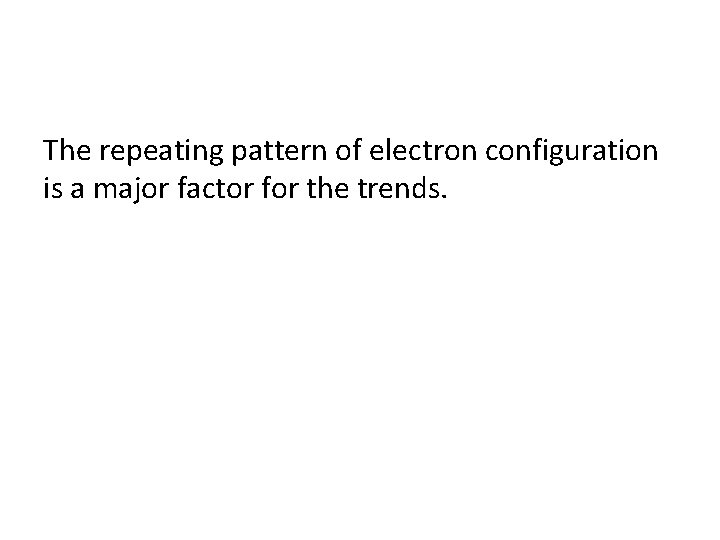The repeating pattern of electron configuration is a major factor for the trends.Electron Configuration and Valence As noted earlier, in each family the general ending electron configuration is the same. The alkali metals all have 1 electron in the “s” orbital. The alkaline earth metals all have 2 electrons in the “s” orbital.Valence Electrons For the main group (s and p block), the number of valence electrons starts at 1 and ends with 8 (octet, noble gas configuration). Every column has the same number of valence electrons. Column 1 = 1 valence electron (Alkali) Column 2 = 2 valence electrons (Alkaline Earth) Column 13 = 3 valence electrons Column 14 = 4 valence electrons Column 15 = 5 valence electrons Column 16 = 6 valence electrons (Chalogens) Column 17 = 7 valence electrons (Halogens) Column 18 = 8 valence electrons (Noble Gases)Practice How many valence electrons does: Ca As I Ne 2 5 7 8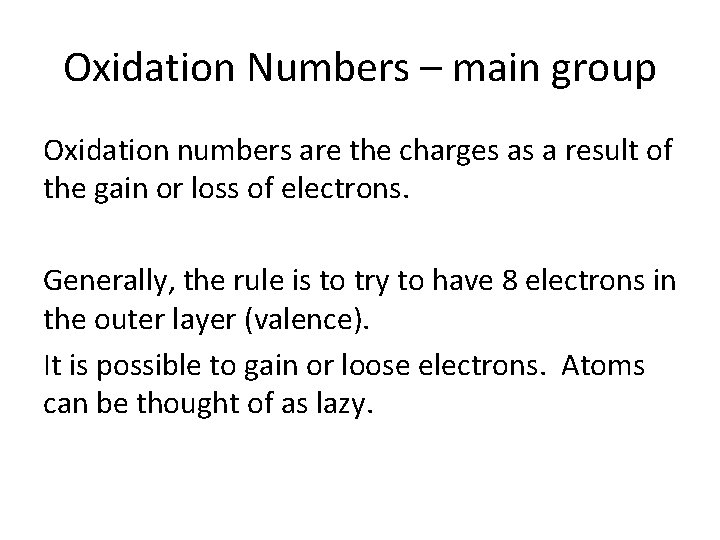Oxidation Numbers – main group Oxidation numbers are the charges as a result of the gain or loss of electrons. Generally, the rule is to try to have 8 electrons in the outer layer (valence). It is possible to gain or loose electrons. Atoms can be thought of as lazy.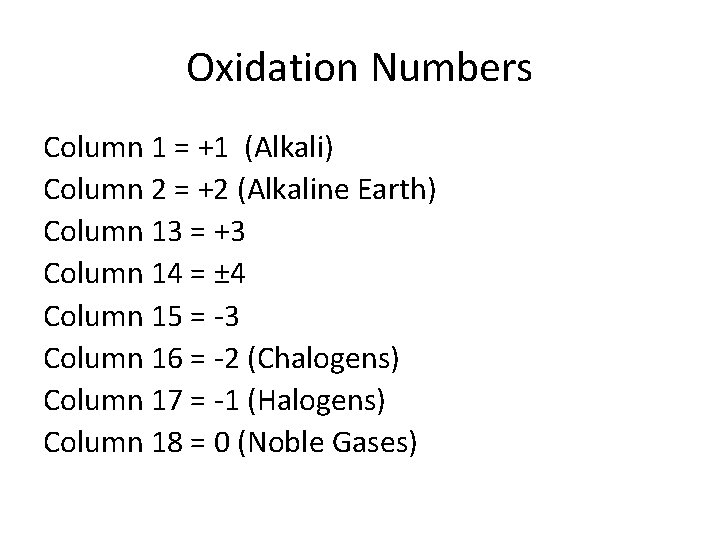Oxidation Numbers Column 1 = +1 (Alkali) Column 2 = +2 (Alkaline Earth) Column 13 = +3 Column 14 = ± 4 Column 15 = -3 Column 16 = -2 (Chalogens) Column 17 = -1 (Halogens) Column 18 = 0 (Noble Gases)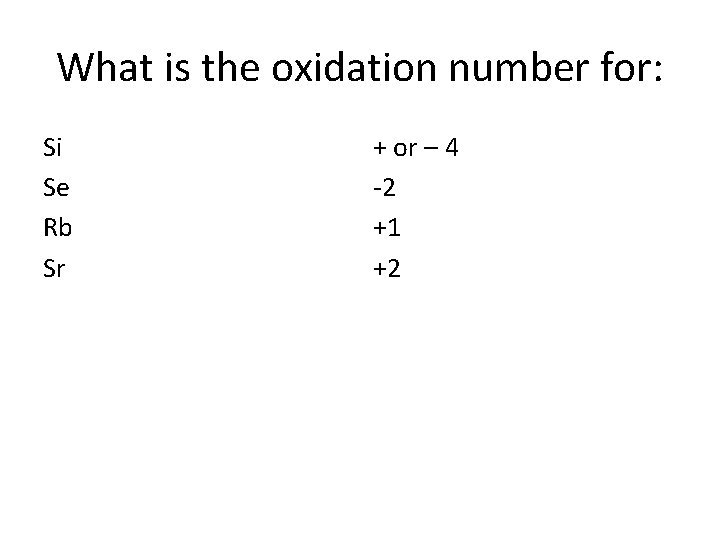What is the oxidation number for: Si Se Rb Sr + or – 4 -2 +1 +2Atomic Radius One of the many trends that emerged from the modern periodic table is atomic radius.First as you travel down the column (family), the radius increases. Why? More layers of electrons on each level which block some of the positive charge pull from the nucleus.The atomic radius decreases across a period. Why? As you travel across the periodic table (in a period), the number of layers does not change. As you move from one box to the next box, the number of protons increases, and the electrons are going to the same outer energy level.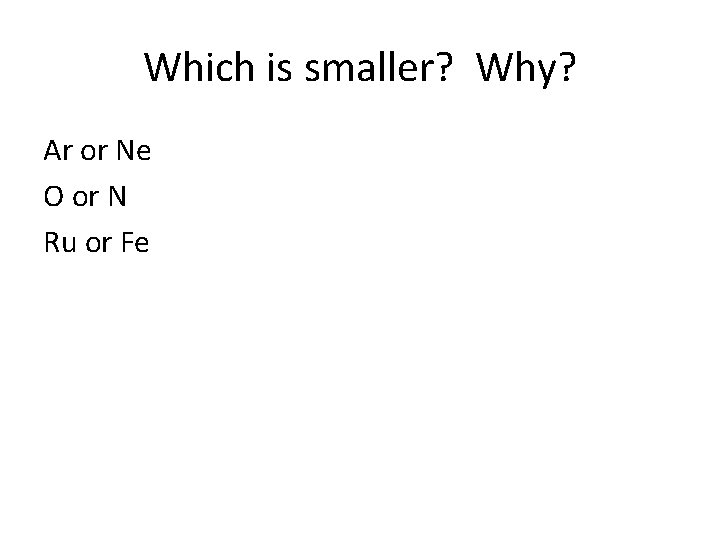Which is smaller? Why? Ar or Ne O or N Ru or Fe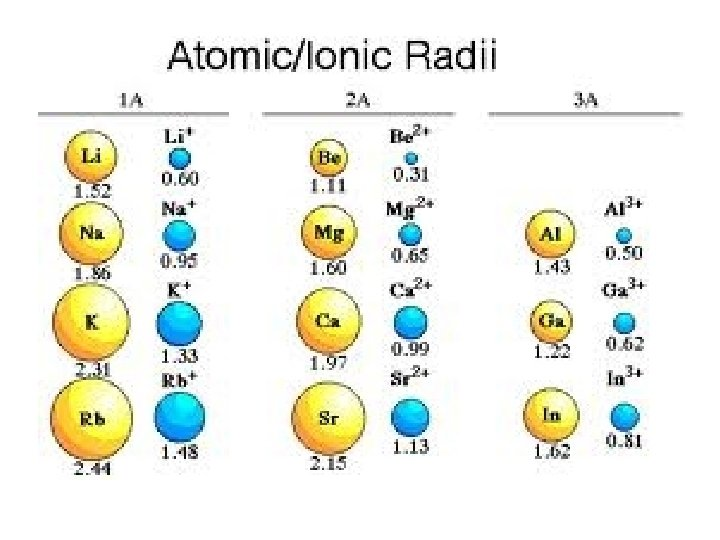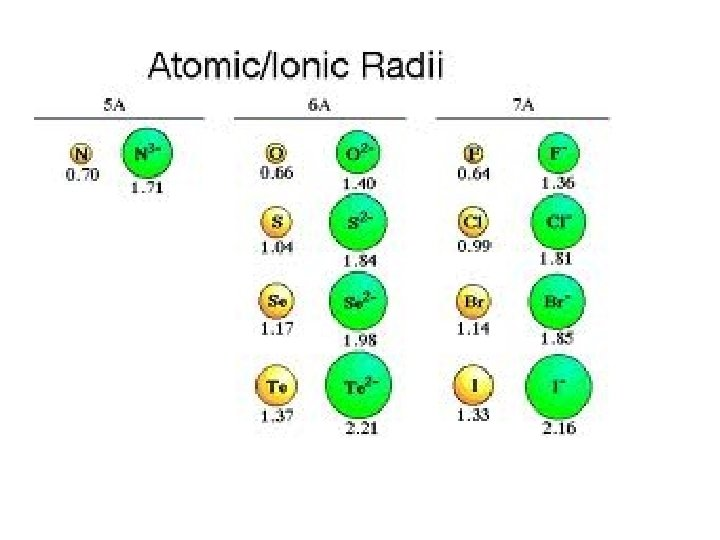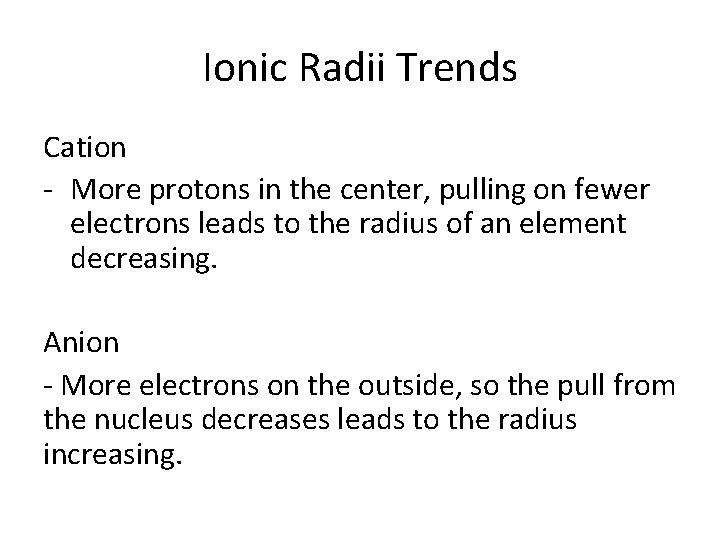Ionic Radii Trends Cation - More protons in the center, pulling on fewer electrons leads to the radius of an element decreasing. Anion - More electrons on the outside, so the pull from the nucleus decreases leads to the radius increasing.Which is larger? Why? F or FCa or Ca 2+ K or K+